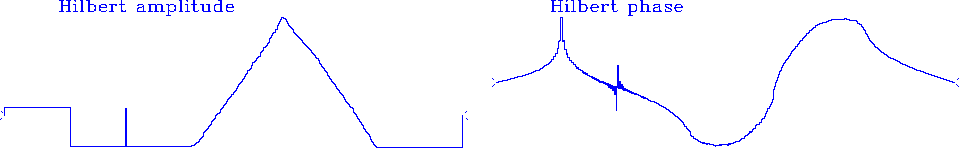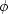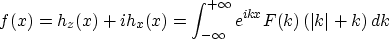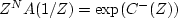Next: A BUTTERWORTH-FILTER COOKBOOK Up: SPECTRAL FACTORIZATION Previous: Pathological examples

## Relation of amplitude to phase

As we learned from equation (19), a minimum-phase function is determined completely from its spectrum. Thus its phase is determinable from its spectrum. Likewise, we will see that, except for a scale, the spectrum is determinable from the phase.

So far we have not discussed the fact that spectral factorization implicitly uses Hilbert transformation. Somehow we simply generated a phase. To see how the phase arose, recall equation (18) and (19):(20)
Where didcome from? We took Uk + i 0 to the time domain, obtaining ut. Then we multiplied ut by a real-valued step function of time. This multiplication in the time domain is what created the phase, because multiplication in the time domain implies a convolution in the frequency domain. Recall that the Fourier transform of a real-valued step function arises with Hilbert transform. Multiplying in time with a step means that, in frequency, Uk has been convolved withphase-shift filter). So Uk is unchanged and a phase,, has been generated. This explanation will be somewhat clearer if you review the Z-transform approach discussed at the beginning of the chapter, because there we can see both the frequency domain and the time domain in one expression.

To illustrate different classes of discontinuity, pulse, step, and slope, Figure 13 shows another Hilbert-transform pair.hilb2
Figure 13
A Hilbert-transform pair.

## EXERCISES:

1. What is the meaning of minimum-phase waveform if the roles of the time and frequency domains are interchanged?
2. Show how to do the inverse Hilbert transform: given, find u. What is the interpretation of the fact that we cannot get u0?
3. Consider a model of a portion of the earth where x is the north coordinate, +z represents altitude above the earth, and magnetic bodies are distributed in the earth, creating no component of magnetic field in the east-west direction. We can show that the magnetic field h above the earth is represented byHere F(k) is some spatial frequency spectrum.
(a)
By using Fourier transforms, how do you compute hx(x, 0) from hz (x, 0) and vice versa?
(b)
Given hz(x, 0), how do you compute hz(x, z)?
(c)
Notice that, at z = 0,and that F(k) (|k| + k) is a one-sided function of k. With a total field magnetometer we observe thatWhat can you say about obtaining F(k) from this?
(d)
How unique are hx(x) and hz(x) ifis given?
4. Test this idea: write code to factor X(Z) into X(Z)=A(Z)B(Z), where B(Z) is minimum-phase and A(Z) is maximum-phase. Maximum-phase means that ZN A(1/Z) is minimum-phase. First compute.Then remove a linear trend in the phase ofto get N. Then split U with its trend removed into causal and anticausal parts U(Z) = C-(1/Z) + C+(Z). Finally, formand.Next: A BUTTERWORTH-FILTER COOKBOOK Up: SPECTRAL FACTORIZATION Previous: Pathological examples
Stanford Exploration Project
10/21/1998